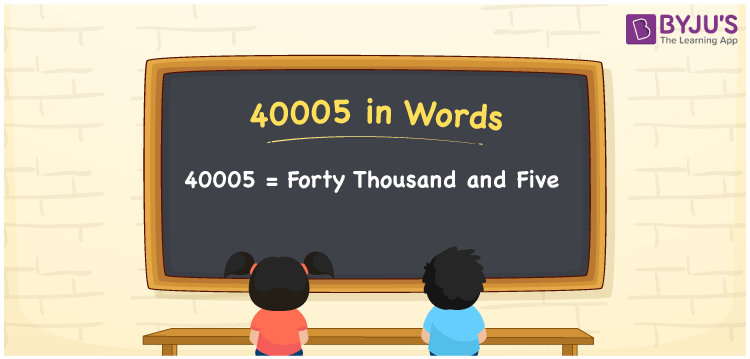# 40005 in Words

40005 in words is Forty thousand and five. Suppose your monthly earnings are Rs. 40005, then you can say, “My monthly earnings is Forty thousand and five rupees”. Here, Forty thousand and five is the number name for 40005. Let’s understand how to write the cardinal number 40005 in words using a place value chart here in this article.

 40005 in words Forty thousand and five Forty thousand and five in Numbers 40005

## 40005 in English Words

We generally write numbers in words using the English alphabet. Thus, we can spell 40005 in English as “Forty thousand and five”.## How to Write 40005 in Words?

As 40005 contains five digits, we should make a place chart with 5 columns as shown below.

 Ten thousands Thousands Hundreds Tens Ones 4 0 0 0 5

This can be written as:

4 × Ten thousand + 0 × Thousand + 0 × Hundred + 0 × Ten + 5 × One

= 4 × 10000 + 0 × 1000 + 0 × 100 + 0 × 10 + 5 × 1

= 40000 + 5

= 40005

= Forty thousand and five

Therefore, 40005 in words is written as Forty thousand and five.

40005 is a natural number that precedes 40006 and succeeds 40004.

40005 in words – Forty thousand and five

Is 40005 an even number? – No

Is 40005 an odd number? – Yes

Is 40005 a perfect square number? – No

Is 40005 a perfect cube number? – No

Is 40005 a prime number? – No

Is 40005 a composite number? – Yes

## Frequently Asked Questions on 40005 in Words

Q1

### Write the number 40005 in words.

We can write the number 40005 in words as Forty thousand and five.
Q2

### How to write 40005 in words on a cheque?

On a cheque, we generally write 40005 in words as “Forty thousand and five rupees only”.
Q3

### Write 40005 in expanded form.

The expanded form of the number 40005, i.e. Forty thousand and five is: 40005 = 40000 + 5 (0r) 40005 = 4 × 10000 + 5 × 1